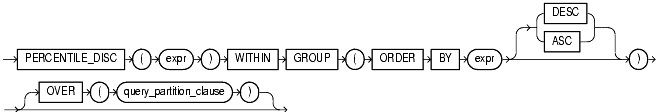## PERCENTILE_DISC

#### Syntax

percentile_disc::=Text description of percentile_disc

 See Also: "Analytic Functions" for information on syntax, semantics, and restrictions of the `OVER` clause

#### Purpose

`PERCENTILE_DISC` is an inverse distribution function that assumes a discrete distribution model. It takes a percentile value and a sort specification and returns an element from the set. Nulls are ignored in the calculation.

The first `expr` must evaluate to a numeric value between 0 and 1, because it is a percentile value. This expression must be constant within each aggregate group. The `ORDER` `BY` clause takes a single expression that can be of any type that can be sorted.

For a given percentile value `P`, `PERCENTILE_DISC` function sorts the values of the expression in the `ORDER` `BY` clause, and returns the one with the smallest `CUME_DIST` value (with respect to the same sort specification) that is greater than or equal to `P`.

#### Aggregate Example

See aggregate example for PERCENTILE_CONT.

#### Analytic Example

The following example calculates the median discrete percentile of the salary of each employee in the sample table `hr.employees`:

```SELECT last_name, salary, department_id,
PERCENTILE_DISC(0.5) WITHIN GROUP (ORDER BY salary DESC)
OVER (PARTITION BY department_id) "Percentile_Disc",
CUME_DIST() OVER (PARTITION BY department_id
ORDER BY salary DESC) "Cume_Dist"
FROM employees where department_id in (30, 60);

LAST_NAME         SALARY DEPARTMENT_ID Percentile_Disc  Cume_Dist
------------- ---------- ------------- --------------- ----------
Raphaely           11000            30            2900 .166666667
Khoo                3100            30            2900 .333333333
Baida               2900            30            2900         .5
Tobias              2800            30            2900 .666666667
Himuro              2600            30            2900 .833333333
Colmenares          2500            30            2900          1
Hunold              9000            60            4800         .2
Ernst               6000            60            4800         .4
Austin              4800            60            4800         .8
Pataballa           4800            60            4800         .8
Lorentz             4200            60            4800          1

```

The median value for Department 30 is 2900, which is the value whose corresponding percentile (`Cume_Dist`) is the smallest value greater than or equal to 0.5. The median value for Department 60 is 4800, which is the value whose corresponding percentile is the smallest value greater than or equal to 0.5.